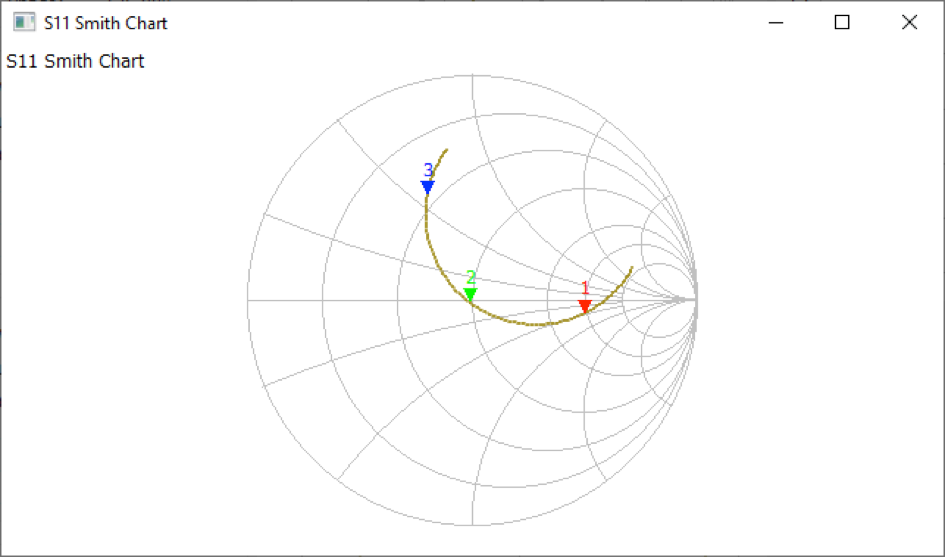Search
• xtgaby

# Magnetic loop 160 meter.

Updated: Aug 12, 2020Calculated coil value of the loop is 6.4 uH as explained in de 40 meter band loop.

Target frequency = 1.858 KHz

Calculated capacity value = 1.100 pF.

I have used in this case 11 meter RG58 with a capacitance of 100 pF / meter.

As you can see on this picture.

I had to change the small loop to insert the signal to a total lenght of 3 meter diameter becomes 1 meter for best SWR figures.Marker 1 is SWR = 3 lower frequency,

Marker 2 is SWR =1,

Marker 3 is SWR = 3 higher frequency.

This means that we have an SWR =3 bandwith of 1.873 – 1.843 = 30 KHz.